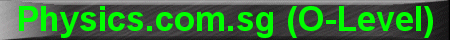(New tips are continually added to these pages.  Check back in a few months' time for more)

TOPIC 11:    Sound

Tip 1:

Echo problems are teachers' favourites in exams because they are usually more challenging. Remember to multiply by a factor of '2' to the distance between the source and the reflecting wall, in order to get the distance traveled by the sound wave.

Example: I stand between two opposite-facing walls separated by 425m and shout.  The time interval between hearing the first echo and second echo is 1.5 seconds.  How far am I standing from each wall?

Solution: Let x be the distance from me to the nearer wall; and y the distance from me to the further wall.  Therefore x + y = 425m

The distance travelled by the first echo was 2x (towards the wall and then back to me), so the time it took was 2x/340 seconds (speed of sound is taken as 340 m s-1).  Similarly, the distance traveled by the second echo was 2y, so the time it took was 2y/340 seconds.  The time interval (1.5 seconds) was the difference between 2y/340 and 2x/340.  Therefore 2y/340 - 2x/340 = 1.5

Equation 1:    x + y = 425

Equation 2:    2y/340 - 2x/340 = 1.5

Two equations, two unknowns.  Solving by simultaneous equation, we get x = 85m and y = 340m.